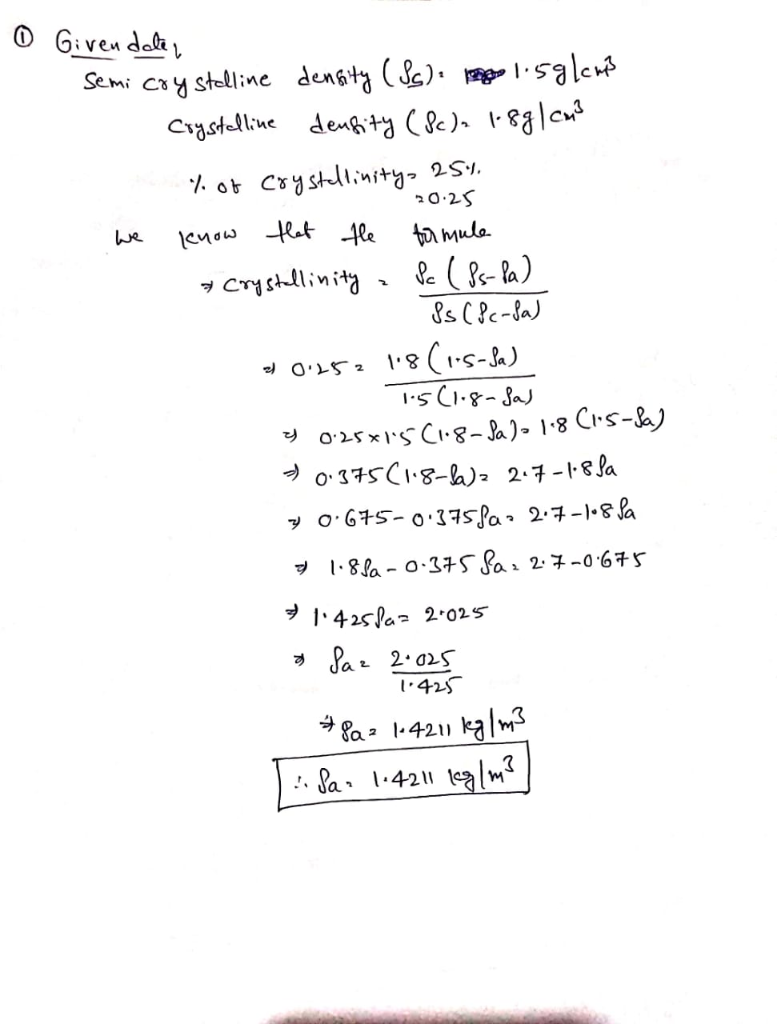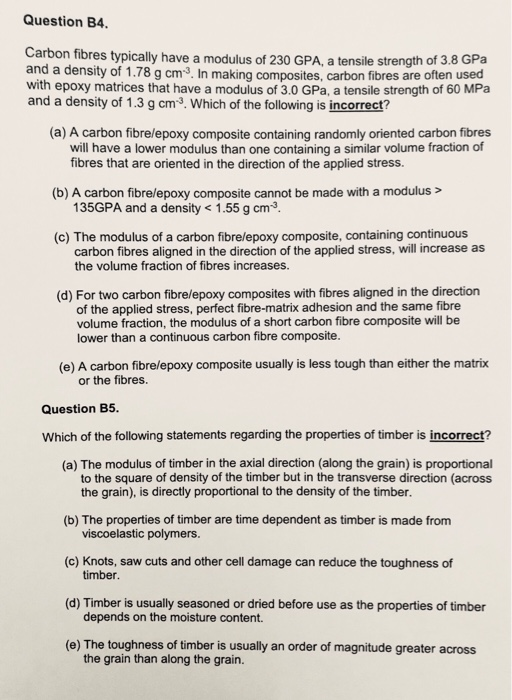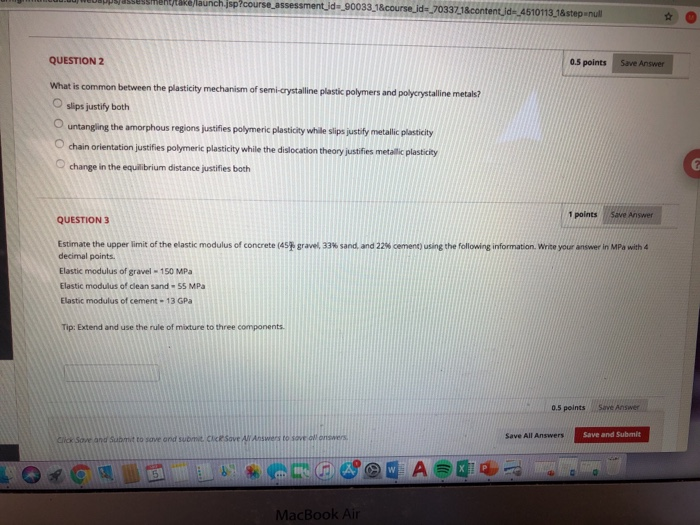# QUESTION 1 A semi-crystalline nylon with a degree of crystallinity of 25% has a density of 1.5 g/cm^3. Nylon has a crystalline density of 1.8 g/cm^3. What is its amorphous density in grams per centi...

QUESTION 1

1. A semi-crystalline nylon with a degree of crystallinity of 25% has a density of 1.5 g/cm^3. Nylon has a crystalline density of 1.8 g/cm^3. What is its amorphous density in grams per centimeters cubed?

1 points

QUESTION 2

1. Which defect is NOT common between metals and polymers?

 tie molecules vacancies screw dislocations interstitial defects

0.5 points

QUESTION 3

1. Which one is not a characteristic of a plastic polymer?

 a. Low stress but very large strains b. Tensile strength is larger than the yield strength c. Tensile strength is lower than the yield strength d. Elastoplastic behaviour

0.5 points

QUESTION 4

1. Elastic modulus of carbon fibers is 260 GPa and the elastic modulus of titanium is 110 GPa. Design a carbon fiber reinforced titanium composite with longitudinal continuous fibers which has a longitudinal elastic modulus of 140 GPa. What is the volume fraction of fibers in percentage? Write your answer without the percentage symbol.

1 points

QUESTION 5

1. What is CORRECT about plastic polymers and ductile metals?

 both become more brittle at high temperatures both have lower tensile strength than the yield stress both become brittle by decreasing the strain rate both fail at the yield strength

0.5 points

QUESTION 6

1. What is the specific strength of a fiber with a density of 7.85 g/cm^3 and tensile strength of 450 MPa? Write your answer in GPa with 4 decimal points.

0.5 points

QUESTION 7

1. Which one is the property of Laminar composites?

 Overall anisotropic Layer-wise anisotropic Overall isotropic Single layered

0.5 points

QUESTION 8

1. For a "linear homopolymer with small molecules" which property is highly likely?

 high density high elastic modulus high hardness low ductility

0.5 points

QUESTION 9

1. Estimate the lower limit of the elastic modulus of concrete (50% gravel, 35% sand, and 15% cement) using the following information. Write your answer in MPa with 4 decimal points.

Elastic modulus of gravel = 120 MPa

Elastic modulus of clean sand = 50 MPa

Elastic modulus of cement = 13 GPa

Tip: Extend and use the rule of mixture to three components.

1 points

QUESTION 10

1. It is aimed to get the highest elastic modulus and strength at every direction in a composite. Which approach should be adopted?

 Randomly disperse the fibers and increase their aspect ratio Randomly disperse the fibers and decrease their aspect ratio Align the fibers and increase their aspect ratio Align the fibers and decrease their aspect ratio

0.5 points

QUESTION 11

1. Which one of the following polymers is more likely to be a thermoplastic?

 linear polymers branched polymers cross-linked polymers network polymer

0.5 points

QUESTION 12

1. Which one is CORRECT about particle-reinforced composites?

 Small particles bear the majority of the load. Large particles do not bear any loads. Dislocation arrest happens by both small and large particles. Both small and large particles have micro interactions with the matrix.

0.5 points

QUESTION 13

1. Which one is correct?

 a. The reinforcements of a composite can be fibers or particles. b. Small particles carry the majority of load in a composite. c. Cermets are composites with ceramic matrix and metallic particles. d. Large particles in a composite is called dispersions.#### Earn Coin

Coins can be redeemed for fabulous gifts.

Similar Homework Help Questions
• ### Question B4 Carbon fibres typically have a modulus of 230 GPA, a tensile strength of 3.8 GPa and a density of 1.78...Question B4 Carbon fibres typically have a modulus of 230 GPA, a tensile strength of 3.8 GPa and a density of 1.78 g cm3. In making composites, carbon fibres are often used with epoxy matrices that have a modulus of 3.0 GPa, a tensile strength of 60 MPa and a density of 1.3 g cm3. Which of the following is incorrect? (a) A carbon fibre/epoxy composite containing randomly oriented carbon fibres will have a lower modulus than one containing a...

• ### QUESTION2 What is common between the plasticity mechanism of semi-cystalline plassic polymers and polycnystalline metalbs? O slips justify both O untangling the amorphous regions justifies polyme...QUESTION2 What is common between the plasticity mechanism of semi-cystalline plassic polymers and polycnystalline metalbs? O slips justify both O untangling the amorphous regions justifies polymeric plasticity while slips justity metallic olasticity 0.5 points Save Answer chain orientation justifies polymeric plasticity while the dislocation theory justifies metalic plasticity chanege inthe equaterium distance jesifits both 1 points Save Answer QUESTION 3 Estimate the upper l mit of the eastK modulus of concrete (45 decimal points Elastic modulus of gravel 150 MPa...

Free Homework App